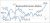# A basic course in number theory

Free!

language English Optional, paid
Category:

This course intends to develop the basics of number theory touching upon many essential points such as the prime number theorem, quadratic reciprocity laws, Gauss’ theorem on the classification of binary quadratic forms, Brahmagupta-Pell equations, to quote a few. This course will enable a student to learn more advanced topics in number theory.

COURSE LAYOUT

Week 1:Factorization of numbers, primes
Week 2:GCD, Euclid’s algorithm, properties of primes
Week 3: Arithmetical functions, examples
Week 4:Dirichlet product, M?o?bius inversion
Week 5:Congruences, Chinese remainder theorem, primitive roots
Week 7: Binary quadratic forms, Gauss’ theory of reduced forms
Week 8:Sums of two squares, sums of four squares
Week 9:Diophantine approximation, theorems of Dirichlet and Liouville
Week 11: Quadratic extensions of rationals, units in the rings of integers
Week 12:Diophantine equations with special reference to Brahmagupta-Pell equation.

## User Reviews

0.0 out of 5
0
0
0
0
0

There are no reviews yet.

×